# Summary & Review: Nand to Tetris Part 1

The Nand-to-Tetris course teaches how to build a computer from primitive Nand gates. The second part teaches how to build a fully fledged High level programming language running the Hack computer built in the first part.

The Nand-to-Tetris course is divided into two parts. Professor Noam Nisan and Shimon Schocken teach the courses. The first part deals with the hardware ranging from a primitive Nand gate to a CPU. The course is divided into 6 projects with increasing level of difficulty.

The second part is focused on teaching Software aspect such as high level programming language which runs on the Hack computer.

The Elements of Computing Systems - Building a Modern Computer from First Principles is the referred book for the course. The book is little cryptic to read if you are not doing the online course. For that, I would suggest another book Code: The Hidden Language of Computer Hardware and Software by Charles Petzold which explain the same concepts but in detail.

Overall, the course balances theory with hand-on-practice very well and has right level of difficulty every week.

The second part requires that you know basics of “object oriented programming” as the Jack programming language in the course is similar to Java.

# Summary

## Week 1

### Big picture

The book is divided into 13 chapters and each chapter is equipped with a project. Also, each chapter builds on the previous chapter.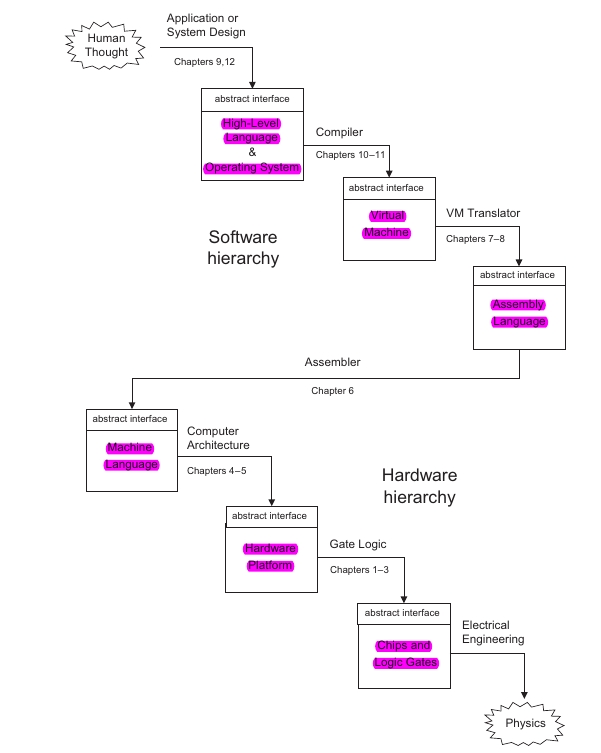Image: Chapter Layout

### Abstraction

Abstraction is dealing with What is not How-to. Every program is itself an abstraction.

In code System.out.printlin("Hello world"), Every character is an abstraction.

Once we implement the details of an abstraction stage, we forget the implementation and just focus on the abstraction.

For example, we implemented an addition function in stage 1. In stage 2, we just focus on calling the function and not worried about how the function is implemented.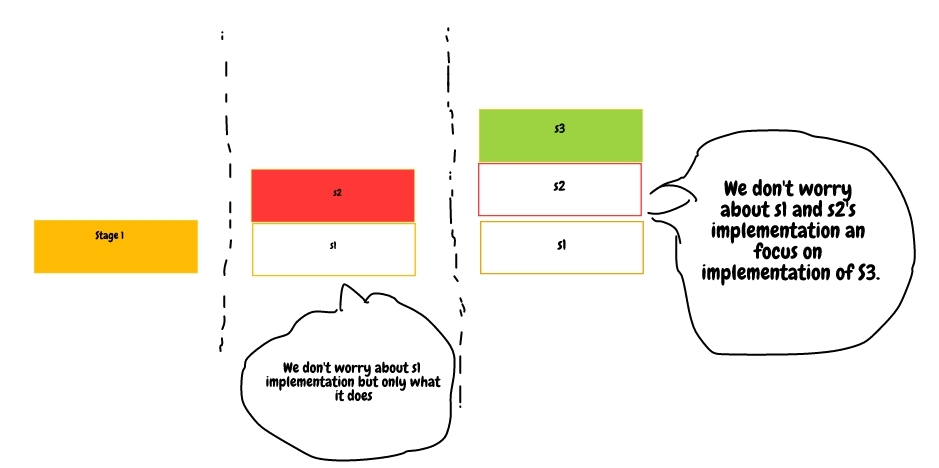The abstraction is build layer by layer

### Separation of Concerns

• Once we implement something, we forget about how-to and just remember what-is

### Layers of Abstraction

• Question: Why do we start at Nand gate?.
• Below the level of Nand gate, there is solid state physics and Electrical engineering.
• Computer Science deals with the level of Nand gate and above.

### The process of building a chip

1. Truth Table
1. Logic diagram/Chip diagram
1. HDL program
1. Testing

#### Example: Building a Xor chip

1. Truth Table
000
011
101
110
1. Chip diagram
• Use the karnaught map to find the number of gates required to build the logical structure.
•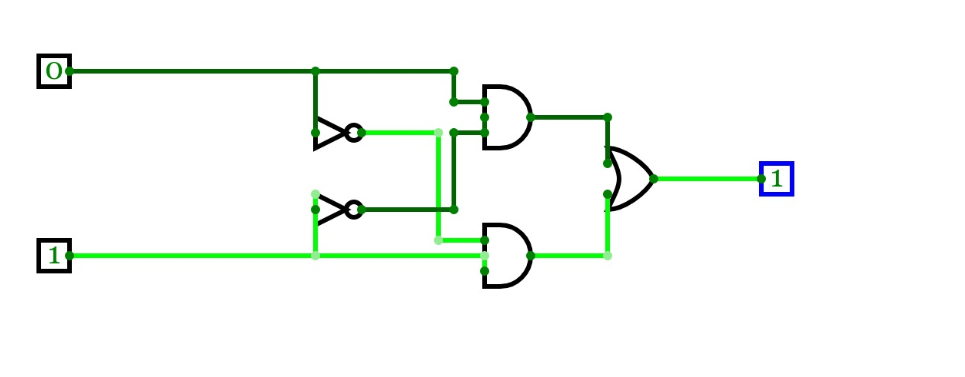1. Write a HDL program
/**
*  Exclusive-or gate: true if either a is true and b is false, or
*  a is false and b is true; false otherwise.
*/

CHIP Xor {
IN a, b;
OUT out;

PARTS:
Not (in=a, out=nota);
Not (in=b, out=notb);
And (a=a, b=notb, out=x);
And (a=nota, b=b, out=y);
Or (a=x, b=y, out=out);
}


1. Test Scripts
• Debug, test and complete.

Gates to build
NANDAND16
NOTOR16
ORMUX16WAY
XOROR8WAY
MUXMUX4WAY16
DMUXMUX8WAY16
NOT16DMUX4WAY
DMUX8WAY

### Boolean Values

The computer works on boolean logic. The boolean consists of only two kinds of state. True and false, 0 and 1

### Truth Table

• All possible values of Input and Output.

### Boolean Identities

Commutative(x and y) = (y and x)(x or y) = (y or x)
Associative(x and (y and z))= ((x and y) and z)(x or(y or z))= ((x or y) or z)
Distributive(x AND (y OR z) = ((x AND y )OR(x AND z )(x OR (y AND Z)) = ((x OR y )AND(x OR z )
DeMorganNOT(x AND y) = NOT(x) OR NOT(y)NOT(x OR y) = NOT(x) AND NOT(y)

### Why Nand Gate

• The Nand gate can replace NOT,AND, OR gates.

#### Building Not from NAND

• NOT gate truth table.
inOUT
01
10
• A NAND gate with a single source connected to both inputs resembles a NOT gate.
001
110
x NAND y = NOT(x AND Y)
x NAND x = NOT (x AND x)
Here x AND x = x
so,
X NAND x = NOT(x)

Similarly, NAND gate can be utilized to construct AND, OR gates.


### Interface vs Implementation

• There must be one interface but there can be many implementations.

#### Circuit Implementations

• AND, OR gates implementation at circuit level
•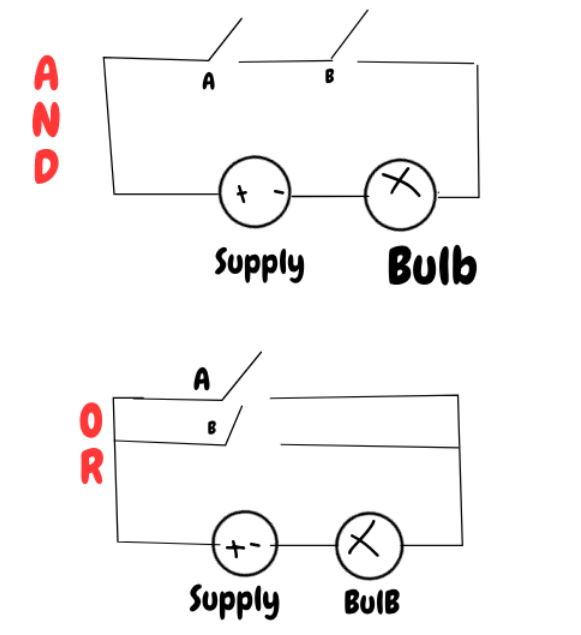### HDL guide

• HDL is hardware description language.
• Readability matters so name internal wires sensibly.
• functional language.
• You can write code in any order.

### Multi-bit Bus

• Multi-bit bus allows to manipulate a group of bits together.
•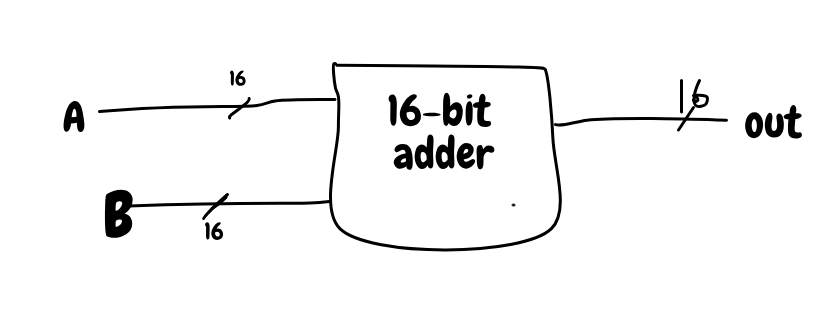• It convenient to think of 16 bit as an array or group. (single unit)
• However, its implemented as 16 wires.
• So A+B is basically addition of two 16-bit numbers.
• The grouping is called a bus.

### Multiplexer

•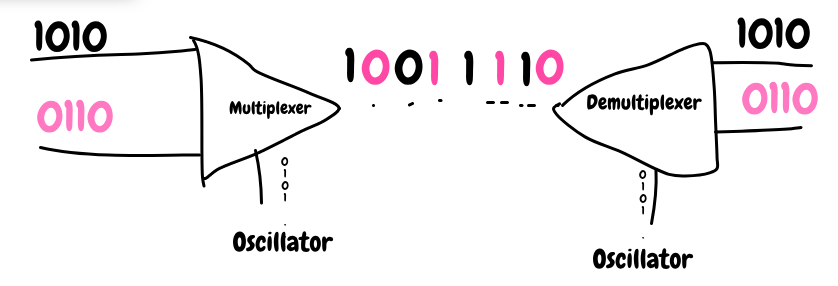• Multiplexer transmit two sources on a single line.
• The source and destination does not need to synchronize with master clock.
• Each can have their own oscillator.

### 16Bit 4 way Multiplexer

• 4 way implies that there are 4 input sources.
• 16 bit indicates that each input is 16 bit.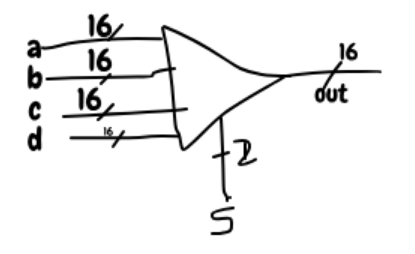### Number representation

• 8 bit = 2^8 bits = 256
• we need to represent range of positive and negative numbers.
•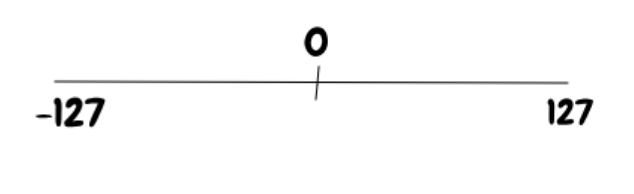### Decimal to Binary

99 =>   64 - 32 - 16 - 8  - 4 - 2  -  1
_________________________________
|64 + 32|                 |    |
------                  |     |
|                     |     |
96 -------------------|2|    |
|     |
98---|1|
|
99
________________________________
1 -  1 -  0 -  0  -  0 - 1 -  1
________________________________



## Week 2

The whole point to represent number is to manipulate it.

The addition is similar to decimal
1 1 1 1
0 0 0 1
-------
1  0 0 0 0    --> The sum
^  -------
|
This is the carry bit which is ignored if overflow.


### overflow

Computer ignores the overflow bit as it cannot be stored.

-> Example: Suppose we add two 8 bit numbers. Then, if addition produces a 9 bit, then it is ignored as we cannot store it in an 8-bit output.

A half adder has two inputs and adds them providing sum and carry.

ABSumCarry
0000
0110
1010
1101

The sum column output is same as XOR The carry column output is same as AND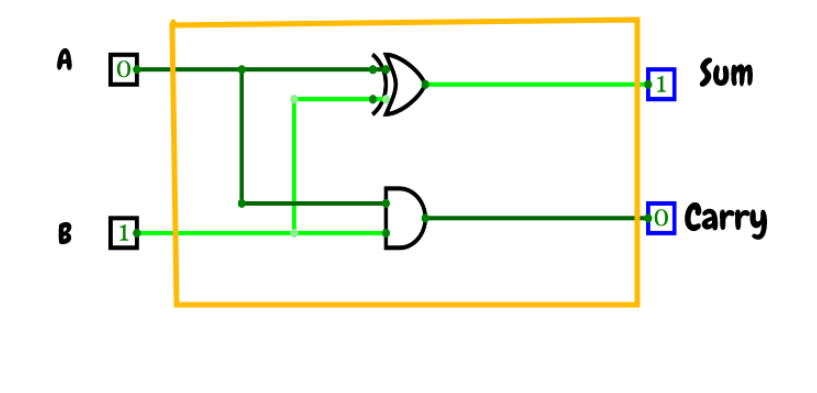ABCSumCarry
00000
00110
01010
01101
10010
10101
11001
11111

#### Sum

= $\overline{\rm a}\overline{\rm b}c+$ $\overline{\rm a}b\overline{\rm c}+$ $a\overline{\rm b}\overline{\rm c}+$ $abc$

= $\overline{\rm a}[\overline{\rm b}c+b\overline{\rm c}]+$ $a[bc+\overline{\rm b}\overline{\rm c}]$

#### Carry

= $\overline{\rm a}bc+a\overline{\rm b}c+ab\overline{\rm c}+abc$

= $c[\overline{\rm a}b+a\overline{\rm b}] +ab[c+ \overline{\rm c}]$

=$ab+c[\overline{\rm a}b+a\overline{\rm b}]$

### Negative numbers

Negative number are represented using 2's complement.

Number$2^2$$2^1$$2^0$$2^0$
00000
10001
20010
30011
40100
50101
60110
70111
81000
91001
101010
111011
121100
131101
141110
151111

### ALU

ALU is arithmetic and logical unit. Arithmetic functions are addition, subtraction, division, multiplication. Logical: And, Xor, OR

• Que: What operations to implement in hardware.
• Depends on tradeoff b/w hardware and software.

#### Design of HACK ALU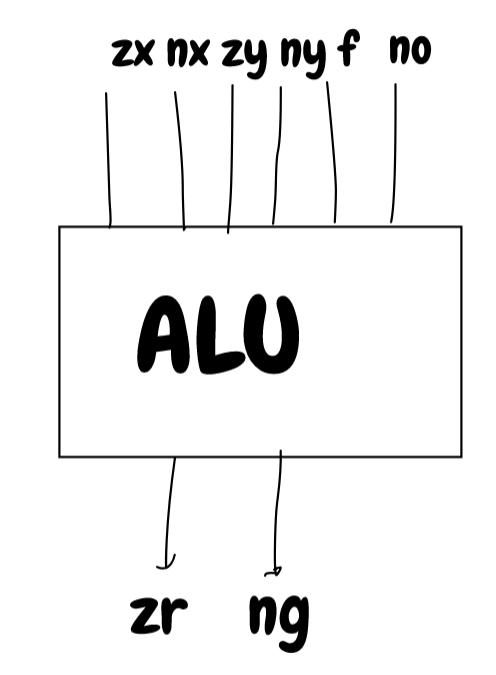zxnxzynyfnoout
y,zx=1

## Week 3: Sequential Logic

Sequential logic includes the concept of time. Sequential logic allows to

1. Reuse hardware
2. Output is function of previous output.

### Time

What do we want time for.

• Primarily for reusing hardware.
• State
• Remember things.
• Eg. Counter, memory.
• Speed: We need to make sure that we don’t ask computer to perform beyond its max speed.

### The Clock

• Analog time is continuous.
• We convert it to discrete time.
• Then, we adjust time cycle to compensate for electrical delays.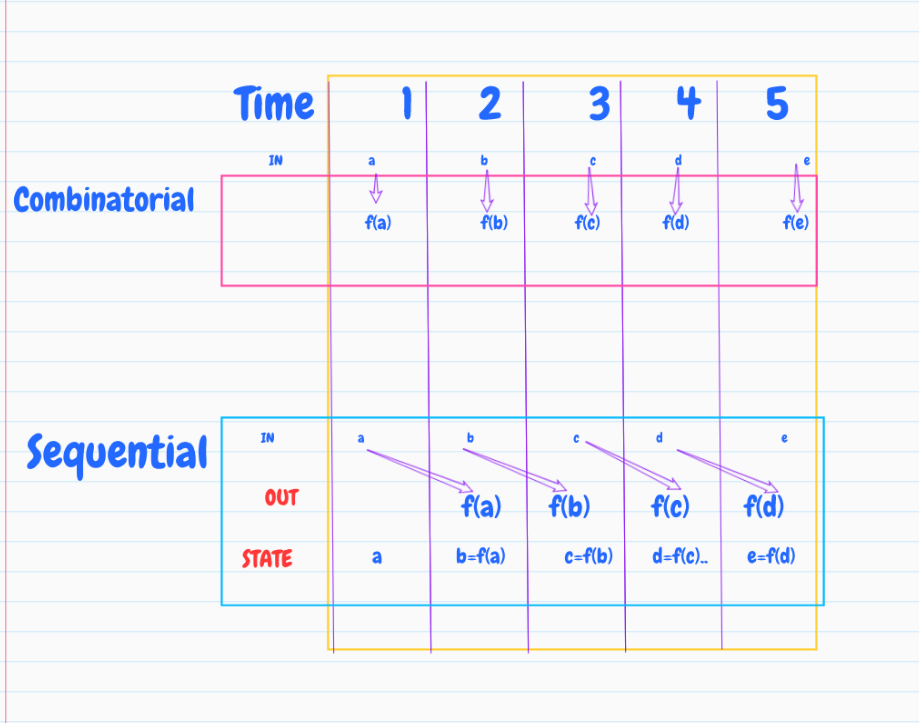#### Electrical signal delay

•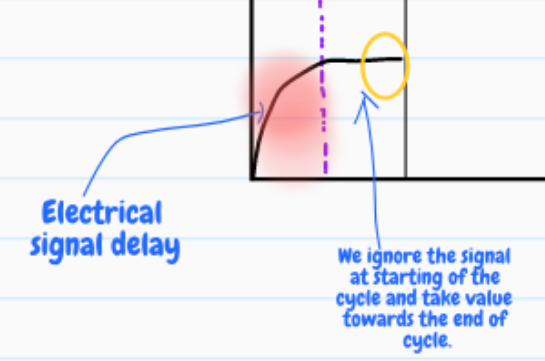• Voltage is continuous(like wave).
• It takes time to change.
• So, we ignore the signal towards start of the cycle.
• Output takes even more time.
• Due to the physical gates delay.
• To counter these, the clock cycle speed is set to allow enough time for both the scenarios.

#### Combinational vs Sequential

• Combinational output depends on input.
• Sequential output depends on previous output(state).
•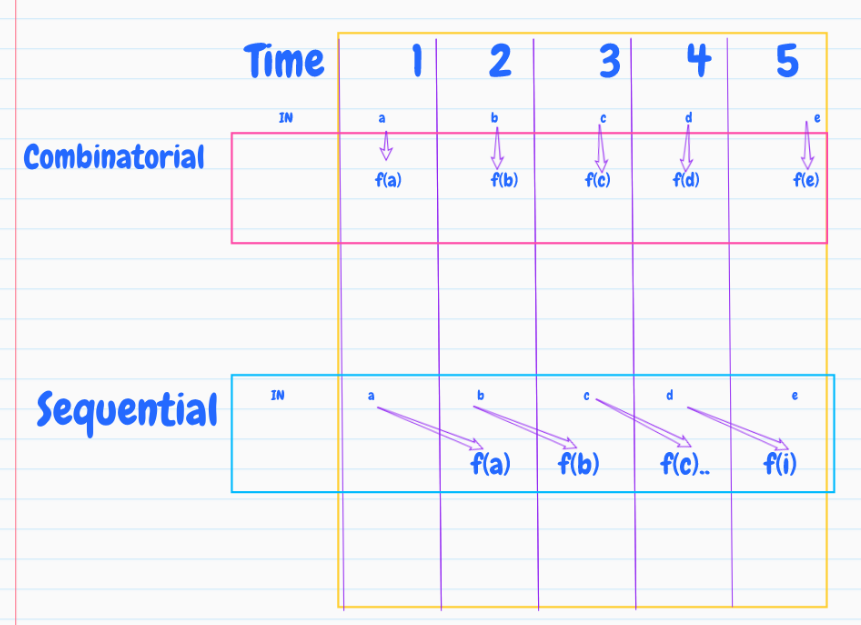### Clocked data flip flop

• SR latch is used to remember previous state.
• It’s built from two Nand gates.
• The previous state can be 0 or 1.
• We need to flip the state.

### 1 Bit Register

load(t-1) -> out(t) = in(t-1)
else       -> out(t) = out(t-1)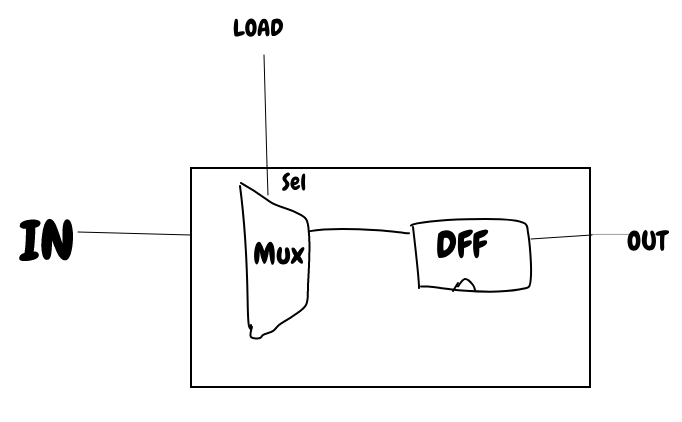### Ram

• Ram is a sequence of n-addressable registers.

At any time, only 1 register is selected.

• k=$log_2{n}$
•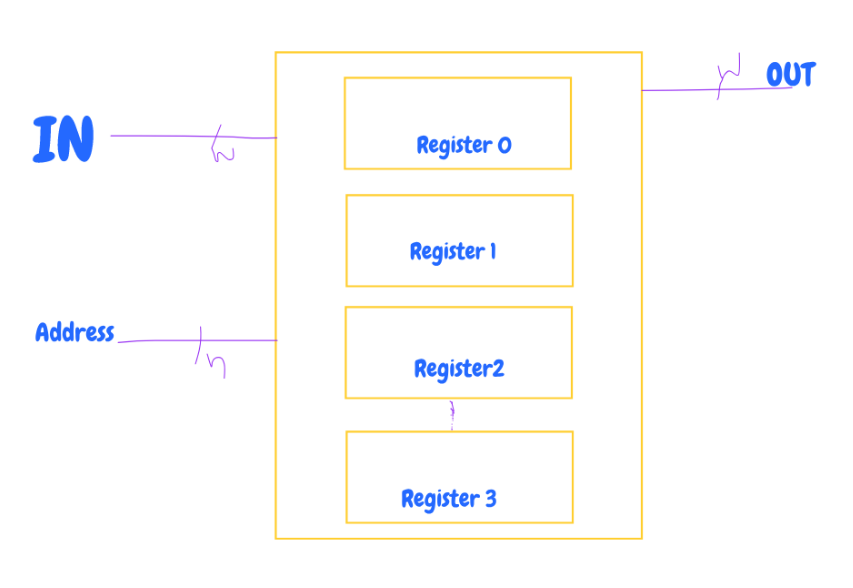### 16 BIT RAM chip family

Namenk
RAM883
RAM64646
RAM5125129
RAM4K409612
RAM16K1638414
• RAM is called Random access memory as you can access random register in same time.

### Counter

• A program counter is useful for 3 scenarios.
1. Increment instruction
1. Go to a specific instruction(JUMP)
1. Reset to 0.

#### Control settings

1. fetch: fetch the first instruction -> pc=0
1. goto: goto n instruction -> pc=n
1. next: fetch the next instruction -> pc++
Reset = 1, out(t+1) = 0
Inc = 1, out(t+1) = out(t) + 1
Load = 0, Inc = 0 (default), out(t+1) = out(t)



### Ram8

• The selector has 3 bits.
s2s1s0
000
001
010
011
100
101
110
111
• Utilize DMux to distribute load to the right register using address bits.

Similarly, Ram64, Ram512 are constructed.

## Week 4

Machine language is low level language.

• It is the interface between hardware and software.

### Universality

Alan Turing theorized a machine with notion of what can be computed. Universal Turing machine - can act like any other Turing machine given the correct program.

• Mnemonics
• Symbolic machine language.
• Example. Add R1 where Add is mnemonic

### Memory Hierarchy

• Accessing big memory for saving each computation is time consuming.
1. time consuming due to data transfer from CPU to memory and vice versa.

The solution is Memory hierarchy which has faster memory such as registers near the CPU and stores the computation results there,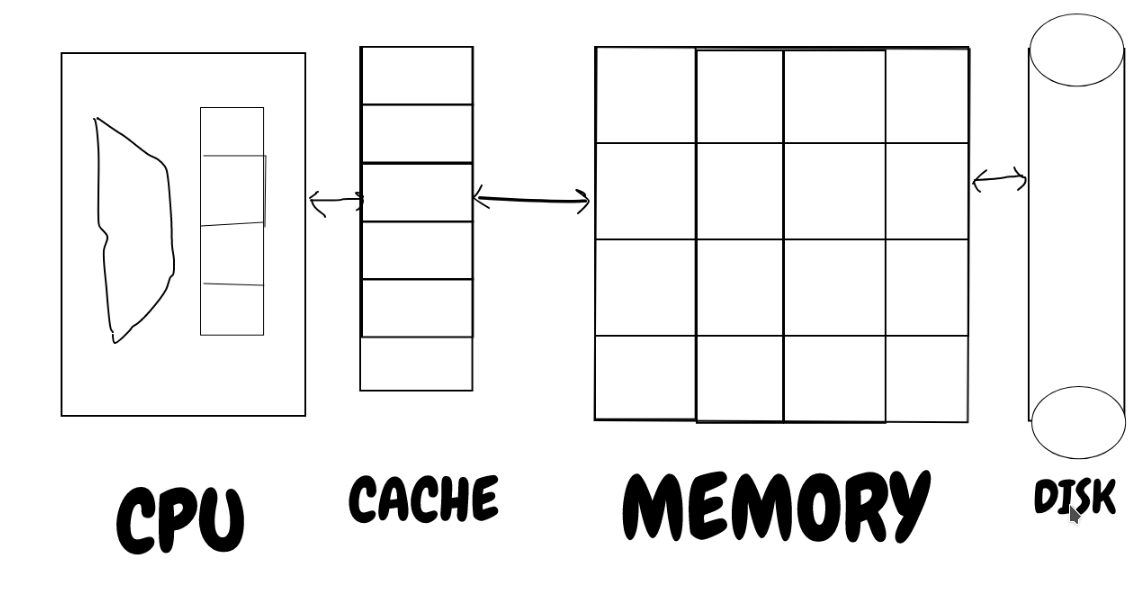### Flow Control

• conditional jump
• The jump is based on the condition.
• For eg. Jump if R1 is not equal to 0
• Unconditional Jump.

### Hack Computer: Hardware

•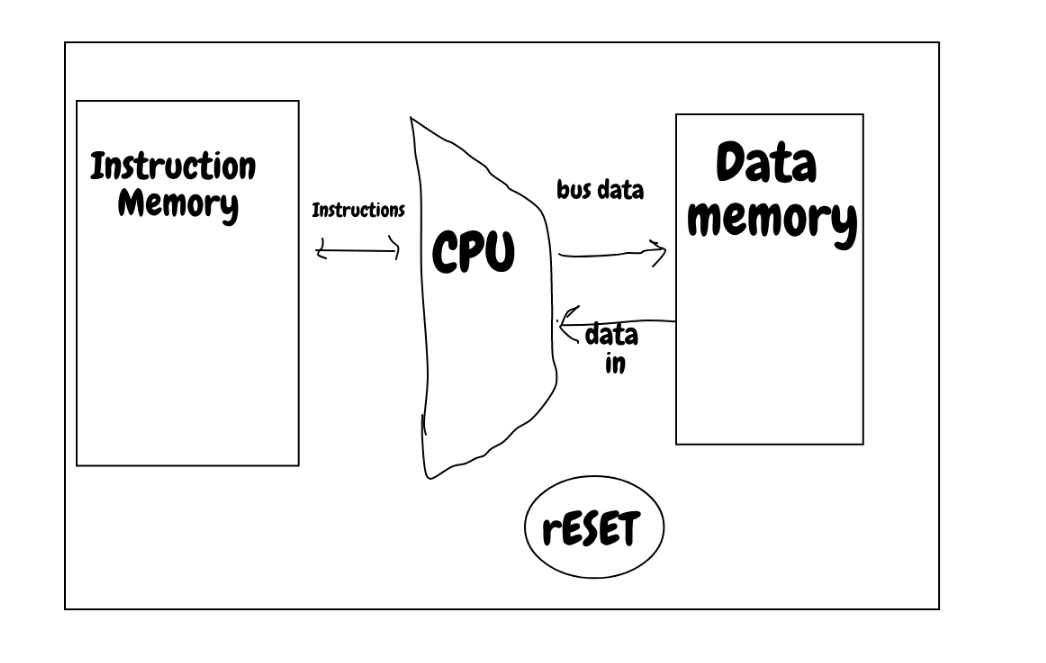### Screen

• Screen is assigned a specific area in the memory called screen memory map.
•• Physical screen is continuously refreshed many times to update the content.

#### How does the program show output

•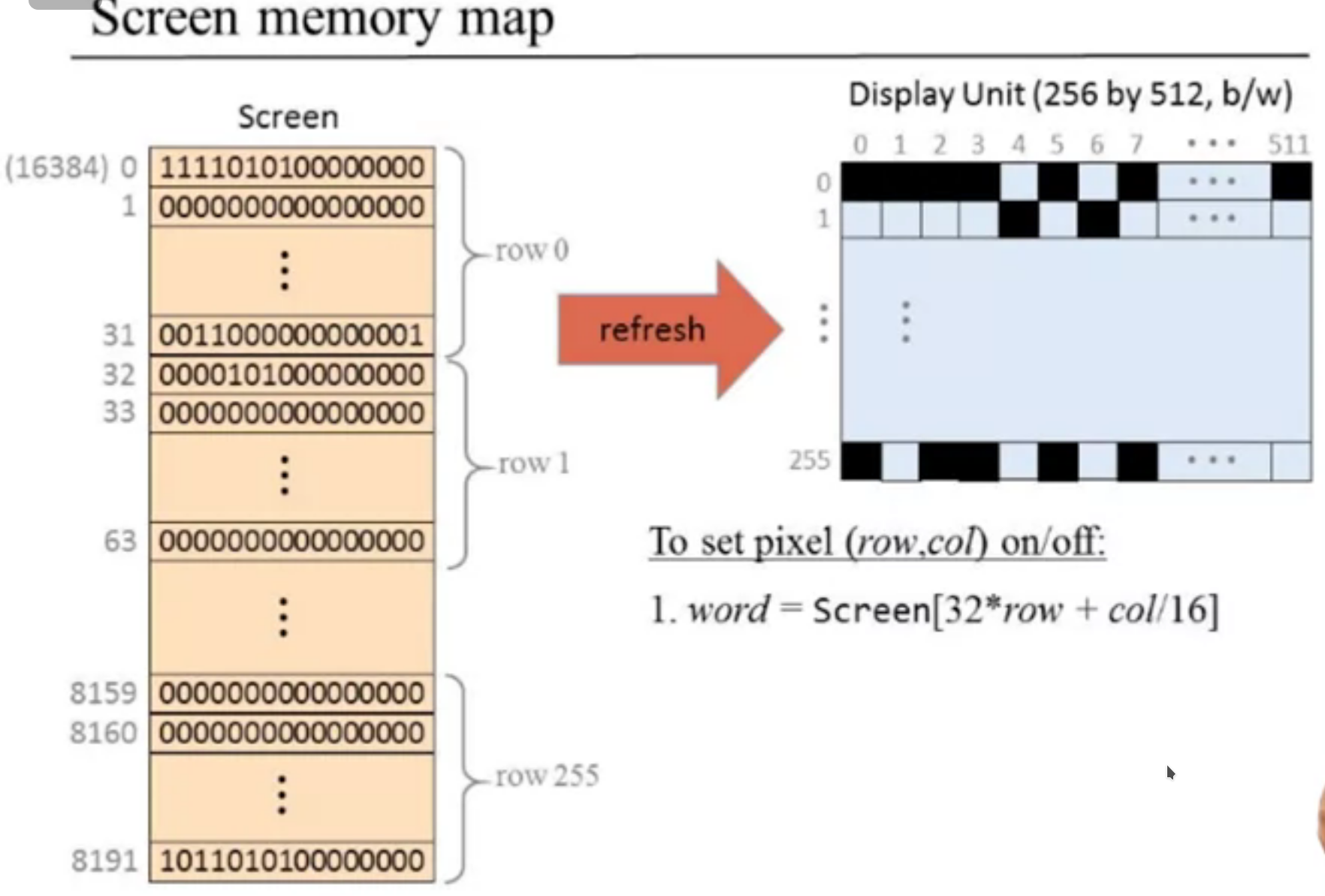• The software driver for the screen updates the screen memory map area.

### Keyboard

• Pressed key’s scan code travels to keyboard memory map.
• It is a 16 bit register.

### NOP Slide attack

• The program if not terminated can run instructions put by a hacker.

#### How to terminate properly.

• Computers never stand still.
• So, to end a program, put an infinite loop at the end of the program.

### Built-in symbols

• We use symbols in machine language to make it easier to read.

## Week 5: Computer Architecture

### Data flow

• 3 types of information passes through the system.
1. Data